﻿ An Elementary Proof of <img src=image/tit1.png></img> and a Recurrence Formula for ζ(2<i>k</i>)Publications are Open
Access in this journal
Article Versions
Export Article
• Normal Style
• MLA Style
• APA Style
• Chicago Style
Research Article
Open Access Peer-reviewed

### An Elementary Proof ofand a Recurrence Formula for ζ(2k)

F. M. S. Lima
Turkish Journal of Analysis and Number Theory. 2017, 5(4), 143-145. DOI: 10.12691/tjant-5-4-5
Received May 27, 2017; Revised June 27, 2017; Accepted June 27, 2017

### Abstract

In this note, a series expansion technique introduced recently by Dancs and He for generating Euler-type formulae for odd zeta values ζ(2k+1), ζ(s) being the Riemann zeta function and k a positive integer, is modified in a manner to furnish the even zeta values ζ(2k). As a result, we find an elementary proof of, as well as a recurrence formula for ζ(2k) from which it follows that the ratio ζ(2k)/π2k is a rational number, without making use of Euler's formula and Bernoulli numbers.

### 1. Introduction

For real values of,, the Riemann zeta function is defined as. In this domain, this series converges according to the integral test.{1} For,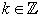,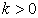, Euler (1740) did find that 4(1)

where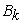is the k-th Bernoulli number, i.e. the rational coefficient of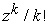in the Taylor series expansion of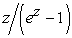,. As a consequence, since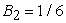one has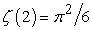, which is the Euler solution to the Basel problem (see Ref. 2 and references therein).

In Ref. 3, Dancs and He (2006) introduced a series expansion approach to derive Euler-type formulae for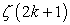. On noting that their approach could be modified in a manner to furnish similar formulas for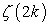, here in this note we show that the change of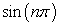by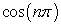in the Dancs-He initial series in fact yields a series expansion which can be reduced to a finite sum involving only even zeta values. From the first few terms of this sum, we have found an elementary proof ofand a recurrence formula for. The proofs are elementary in the sense they do not involve complex analysis, Fourier series, or multiple integrals.{2}

### 2. Elementary Evaluation of ζ(2)

For any realand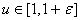, we begin by taking into account the following Taylor series expansion considered by Dancs and He in Ref. 3:(2)

which converges absolutely for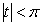.

From the generating function for the Euler polynomial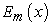, i.e.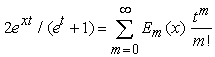, it is clear that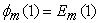, for all nonnegative integer values of m. For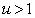, we have(3)

Let us take this series as our definition of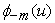,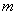being a positive integer. Therefore(4)

for all integer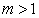.

Now, let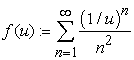be an auxiliary function, with u belonging to the same domain as above. Since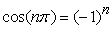, thencan be written in the form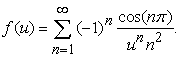On expanding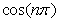in a Taylor series, one finds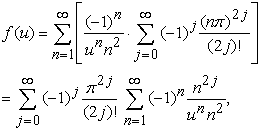in which the change of sums justifies by Fubini's theorem. By writing the last series in terms of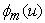, one has(5)

This is enough for the derivation of our first result.

Theorem 1 (Short evaluation of ζ(2))..

Proof. By taking the limit ason both sides of Eq.(5), one has(6)

which, in face of the value ofstated in Eq.(4), implies that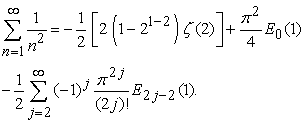(7)

Since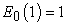andfor all, the right-hand side of this equation reduces to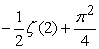,{3} which implies that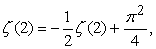and then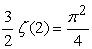.

### 3. Recurrence Formula for ζ(2k)

Interestingly, our approach can be easily adapted to treat higher zeta values by changing the exponent of n from 2 to 2k. The result is the following recurrence formula for even zeta values.

Theorem 2 (Recurrence for ζ(2k)). For any positive integer k,Proof. We begin by defining. Again, since, we may write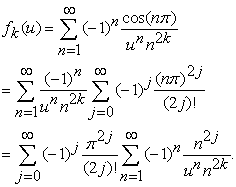(8)

On rewriting the last series in terms of, one hasNow, on substituting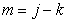in the above series, one finds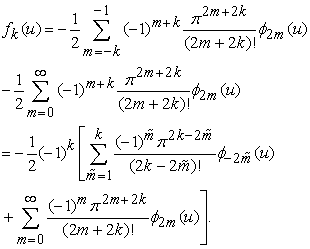(9)

The limit as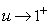, taken on both sides of Eq.(9), yields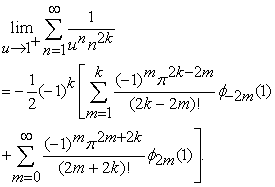(10)

From Eq.(4), one knows that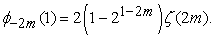For nonnegative values of m, one has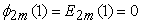, the only exception being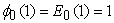. This reduces Eq.(10) to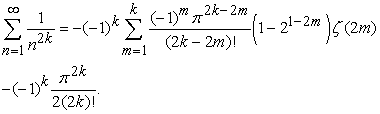By extracting the last term of the sum and isolating ζ(2k), one finds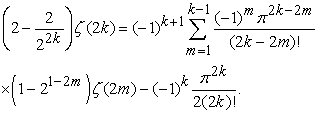A multiplication by 2 on both sides yields the desired result.

The first few even zeta values can be readily obtained from the recurrence formula in Theorem 2. For, the sum in the right-hand side is null and our recurrence reduces to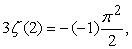which simplifies to, in agreement to our Theorem 1. For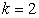, our recurrence yields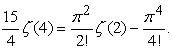By substitutingand multiplying both sides by 4, one finds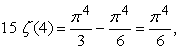(11)

which implies that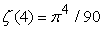.

Note that, by writing the recurrence formula in Theorem 2 in the form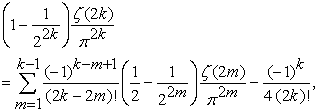(12)

it is straightforward to show, by induction on k, that the ratio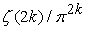is a rational number for every positive integer k, without making use of Euler's formula for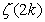, as stated in Eq.(1), and Bernoulli numbers. In fact, this was the original motivation that has led the author to study the properties of the Dancs-He series expansions. The proofs developed here could well be modified to cover other special functions of interest in analytic number theory.

### Notes

1. For s=1, one has the harmonic series, which diverges to infinity.

2. For non-elementary proofs, see, e.g., Refs. [1,5] and references therein.

3. This occurs because E2m(1) = 0 for all positive integer values of m.

### References

  M. Aigner and G.M. Ziegler, Proofs from THE BOOK, 5th ed., Springer, New York, 2014, Chap. 9. In article View Article  R. Ayoub, “Euler and the zeta function,” Am. Math. Monthly 81, 1067-1085 (1974). In article View Article  M. J. Dancs and T.-X. He, “An Euler-type formula for ζ(2k+1),” J. Number Theory 118, 192-199 (2006). In article View Article  L. Euler, “De summis serierum reciprocarum,” Commentarii Academiae Scientiarum Petropolitanae 7, 123-134 (1740). In article  D. Kalman, “Six ways to sum a series,” Coll. Math. J. 24, 402-421 (1993). In article View ArticleThis work is licensed under a Creative Commons Attribution 4.0 International License. To view a copy of this license, visit http://creativecommons.org/licenses/by/4.0/

F. M. S. Lima. An Elementary Proof ofand a Recurrence Formula for ζ(2k). Turkish Journal of Analysis and Number Theory. Vol. 5, No. 4, 2017, pp 143-145. http://pubs.sciepub.com/tjant/5/4/5
Lima, F. M. S.. "An Elementary Proof ofand a Recurrence Formula for ζ(2k)." Turkish Journal of Analysis and Number Theory 5.4 (2017): 143-145.
Lima, F. M. S. (2017). An Elementary Proof ofand a Recurrence Formula for ζ(2k). Turkish Journal of Analysis and Number Theory, 5(4), 143-145.
Lima, F. M. S.. "An Elementary Proof ofand a Recurrence Formula for ζ(2k)." Turkish Journal of Analysis and Number Theory 5, no. 4 (2017): 143-145.